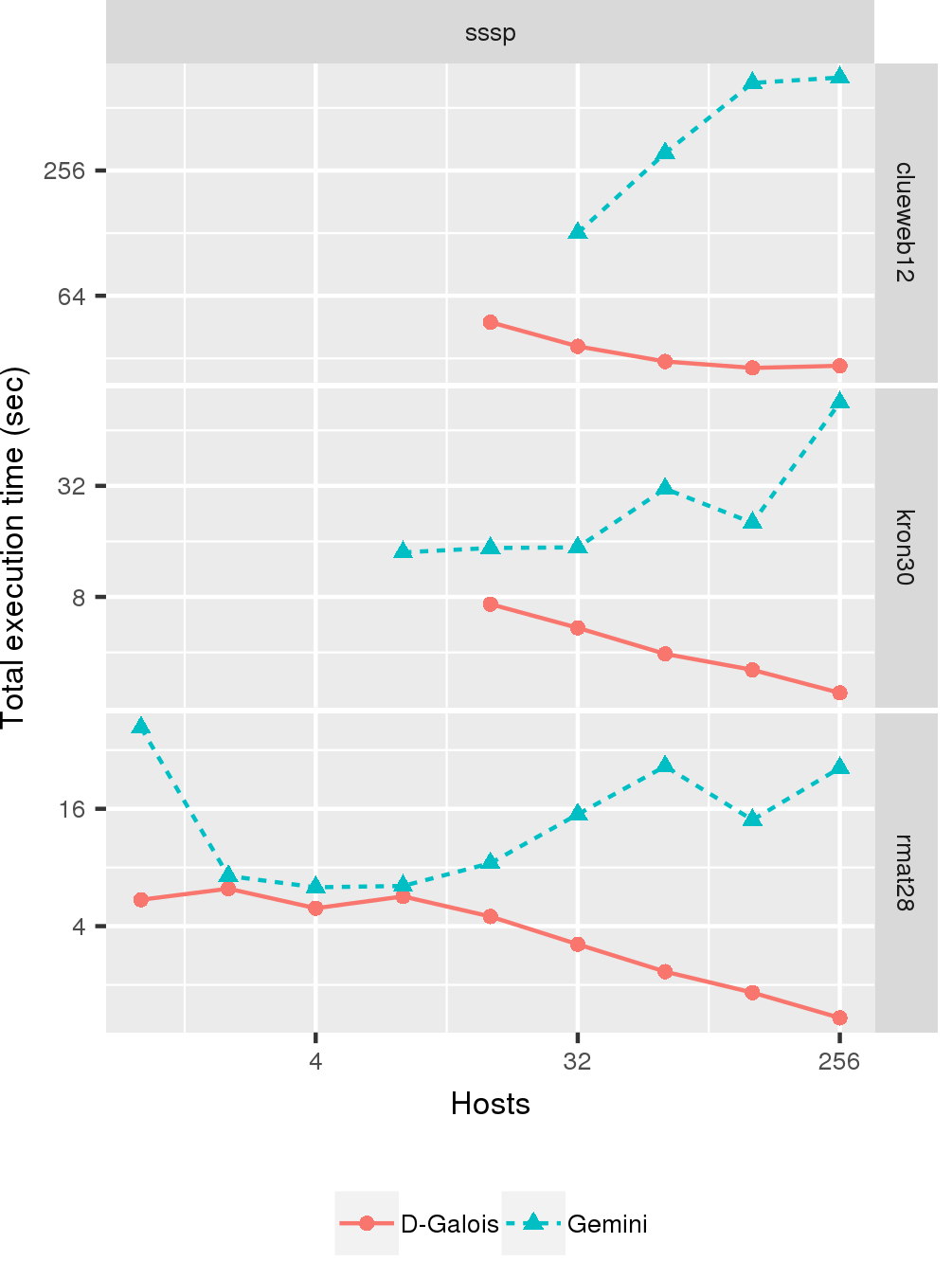# Single-Source Shortest Path

## Application Description

This benchmark computes the shortest path from a source node to all nodes in a directed graph with non-negative edge weights.

## Application Description

We have 2 distributed implementations of SSSP: a push-style and a pull-style. Both are bulk-synchronous parallel (BSP) implementations: execution proceeds in rounds, and synchronization of data among hosts occurs between rounds.

The push-style version checks a node to see if its distance has changed since the last round. If it has, it will update its neighbor's distances using its new distance and the weight of the edge that connects the two. The pull-style version goes over all nodes: all nodes check their in-neighbors, and if a neighbor has a distance that would result in a new shortest path distance once the edge is considered, then a node updates itself with its neighbors' data. Execution of both versions continues until there are no more nodes that are updated in a round.

Psuedocode for the computation step of the 2 implementations follows below:

 1 2 3 4 5 6 7 for (node n in graph) { if (n.distance != n.old_distance) { for (neighbor a of node n) { a.distance = min(n.distance + weight of edge(n,a), a.distance) } } }

Figure 1: Pseudocode for SSSP Push computation

 1 2 3 4 5 6 7 8 for (node n in graph) { for (in-neighbor a of node n) { if (a.distance + weight of edge(a,n) < n.distance) { n.distance = a.distance + weight of edge(a,n) } } } }

Figure 2: Pseudocode for SSSP Pull computation

Synchronization of the distance variable occurs between BSP rounds. A node will take the minimum distance value of all proxies that exist in the system for that node.

## Performance

The graph below shows performance of sssp-push on the TACC Stampede2 cluster's KNL nodes (Intel Xeon Phi 7250, 1.4 Ghz with 96GB DDR4 + 16 GB MCDRAM) running a total of 272 hardware threads from 1 to 256 hosts. We run on 3 graphs: rmat28 and kron30 are randomly generated graphs from the rmat and kron generator, respectively, and clueweb12 is one of the biggest publicly available web-crawl graphs. D-Galois numbers are compared the corresponding benchmark from Gemini, a state-of-the-art distributed graph analytics system.